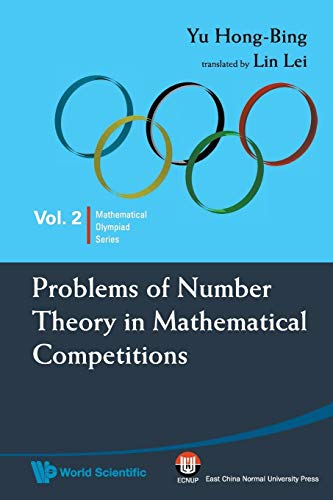Free Shipping on all orders in Australia
Over 7 million books in stock
We aim to be carbon neutral by 2022# Problems Of Number Theory In Mathematical Competitions by Hong-bing Yu (Suzhou Univ, China)

Condition - New
\$74.89
Only 2 left

## Summary

Number theory is an important research field of mathematics. In mathematical competitions, problems of elementary number theory occur frequently. These problems use little knowledge and have many variations. This book introduces some basic concepts and methods in elementary number theory via problems in mathematical competitions.

This book will be specially printed for your order, which means it may take a little longer to arrive (5 days). We do this to cut down on waste & to help protect our planet.

## Problems Of Number Theory In Mathematical Competitions Summary

### Problems Of Number Theory In Mathematical Competitions by Hong-bing Yu (Suzhou Univ, China)

Number theory is an important research field of mathematics. In mathematical competitions, problems of elementary number theory occur frequently. These problems use little knowledge and have many variations. They are flexible and diverse. In this book, the author introduces some basic concepts and methods in elementary number theory via problems in mathematical competitions. Readers are encouraged to try to solve the problems by themselves before they read the given solutions of examples. Only in this way can they truly appreciate the tricks of problem-solving.

Divisibility; Greatest Common Divisors and Least Common Multiples; Prime Numbers and Unique Factorization Theorem; Indeterminate Equations (I); Selected Lectures on Competition Problems (I); Congruence; Some Famous Theorems in Number Theory; Order and Its Application; Indeterminate Equations (II); Selected Lectures on Competition Problems (II).

NLS9789814271141
9789814271141
9814271144
Problems Of Number Theory In Mathematical Competitions by Hong-bing Yu (Suzhou Univ, China)
New
Paperback
World Scientific Publishing Co Pte Ltd
2009-09-17
116
N/A
Book picture is for illustrative purposes only, actual binding, cover or edition may vary.
This is a new book - be the first to read this copy. With untouched pages and a perfect binding, your brand new copy is ready to be opened for the first time